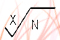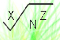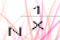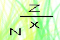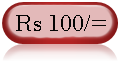Web Development : Starting from Concept, HTML, CSS, Web Site Develpment to Website Launching.
Written by: Sanjay Srivastava
B.Tech (Honours);IIT Kharagpur
PMP ; PMI USA

Class - VIII - Maths:- INDEX

1. Squares & Square Roots
2. Cubes & Cube Roots
4. Profit Loss & Discount
5. Compound Interest
6. Algebraic Identities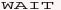7. Polynomials8. Linear Equations9. Parallel Lines10. Types of Quadrilaterals11. Construction of Quadrilaterals12. Circles13. Areas14. Surface Areas15. Volumes16. StatisticsClass - VIII - Maths

## What are exponents (Page 1/4)

Important Tips
(1) The power raised to any rational number is known as it's exponent.
The exponents can be +ve, -ve whole number or fraction.
Here 2 and 1/2 are a positive exponent and -3 is negative exponent.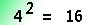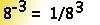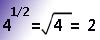(2) Laws of exponents :
If 'X' is a rational number and 'y' & 'z' are rational exponents, then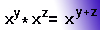&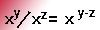Question - Type 1 - Expresses these in exponents form